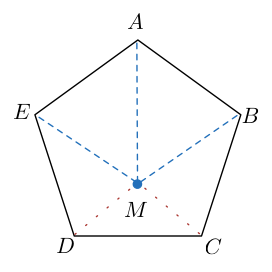# Particular Pentagon Point

Geometry Level 5The point $M$ lies inside regular pentagon $ABCDE$ such that $\angle MBA = \angle MEA = 42^{\circ}$. Find $\angle DMC$.

The above image is not drawn to scale.
$ABME$ need not be a parallelogram.
$BMD$ and $EMC$ need not be straight lines.

×